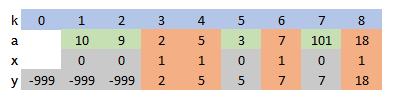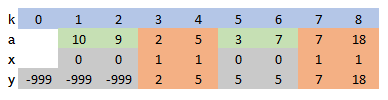## Tuesday, July 14, 2020

### Longest Increasing Subsequence

#### Problem Description

Given a sequence of integers $$a_k$$, find the longest subset of these numbers such that they are increasing .

As an example consider the numbers:

[10,9,2,5,3,7,101,18]
Then the longest increasing subsequence is [2,5,7,101] (or [2,5,7,18]) with length 4.

The purpose of the original question was to develop a dynamic programming algorithm for this.

My question: can we formulate a mathematical programming model for this problem?

#### MINLP formulation for longest non-decreasing subsequence

An obvious choice for the main decision variables is: $x_k = \begin{cases}1&\text{if a_k is selected}\\ 0&\text{otherwise}\end{cases}$ A more difficult issue is: how to make sure that the selected $$a_k$$'s are non-decreasing. One way could be: define an additional variable $$y_k$$ with: $y_k = \begin{cases} a_k & \text{if x_k=1 (pick the selected a_k)}\\ y_{k-1} & \text{if x_k=0 (keep y the same)}\end{cases}$ and make sure $$y_k$$ is non-decreasing. This may not be immediately obvious. BasicallySelect the largest number of non-decreasing values

A quadratic formulation can look like:

\begin{align}\max & \sum_k \color{darkred}x_k \\ & \color{darkred}y_k = \color{darkblue}a_k \cdot \color{darkred}x_k + \color{darkred}y_{k-1} \cdot (1-\color{darkred}x_k) \\& \color{darkred}y_k \ge \color{darkred}y_{k-1} \\&\color{darkred}x_k \in \{0,1\} \end{align}

I used as starting value $$y_0 = -999$$.

The results can look like

----     35 PARAMETER results

k1          k2          k3          k4          k5          k6          k7          k8

a          10           9           2           5           3           7         101          18
x                                   1           1                       1           1
y        -999        -999           2           5           5           7         101         101


Notes:

• The solution is not unique.
• This quadratic model can be solved with global MINLP solvers such as Baron, Couenne and Antigone or a non-convex quadratic solver such as Gurobi.
• The problem can easily be linearized if we can use indicator constraints: \begin{align}& x_k=1 \Rightarrow y_k=a_k\\ & x_k=0 \Rightarrow y_k = y_{k-1}\end{align}
• Otherwise, we can use some big-M constraints: \begin{align} a_k-M(1-x_k)\le &y_k \le a_k+M(1-x_k)\\ y_{k-1}-Mx_k\le &y_k \le y_{k-1}+Mx_k\end{align} Of course, we need to find some reasonable values for the big-M constants.
• For more complex formulation problems, I often try an MINLP formulation first and linearize afterward. I.e. first get the concepts right, then fill in the details.
• The original problem dealt with integers $$a_k$$ and was looking for strictly increasing subsequences. We can fix our model by changing the ordering constraint to $y_k \ge y_{k-1}+x_k$ The results don't change for the above data, but a slightly different data set shows how this can work:Strictly increasing subsequence

We see that from $$a_6 = a_7 = 7$$ only one entry is chosen. The reason is that we add one to $$y_k$$ if a new $$a_k$$ is selected. That means, in that case $$y_k = y_{k-1}+1$$. So no two items with the same value can be selected.
• If we want to use this approach for non-integer numbers $$a_k$$ we can use a bit of a hack: $y_k \ge y_{k-1}+0.001 x_k$

To summarize, the formulation for integer data and strictly increasing subsequences can look like:

\begin{align}\max & \sum_k \color{darkred}x_k \\ & \color{darkred}y_k = \color{darkblue}a_k \cdot \color{darkred}x_k + \color{darkred}y_{k-1} \cdot (1-\color{darkred}x_k) \\& \color{darkred}y_k \ge \color{darkred}y_{k-1}+\color{darkred}x_k \\&\color{darkred}x_k \in \{0,1\} \end{align}

Running the last model (strictly increasing subsequence) on a larger data set shows:

----     34 PARAMETER a  data

k1    18.000,    k2    85.000,    k3    56.000,    k4    31.000,    k5    30.000,    k6    23.000,    k7    35.000
k8    86.000,    k9     7.000,    k10   51.000,    k11  100.000,    k12   58.000,    k13  100.000,    k14   77.000
k15   14.000,    k16   64.000,    k17   16.000,    k18   26.000,    k19   67.000,    k20   44.000,    k21   36.000
k22   36.000,    k23   14.000,    k24   16.000,    k25   59.000,    k26   84.000,    k27   24.000,    k28   67.000
k29   78.000,    k30   31.000,    k31   12.000,    k32   51.000,    k33   17.000,    k34   88.000,    k35   27.000
k36   29.000,    k37   60.000,    k38   73.000,    k39   63.000,    k40   47.000,    k41   42.000,    k42   12.000
k43   32.000,    k44    5.000,    k45   34.000,    k46   19.000,    k47   65.000,    k48   57.000,    k49   77.000
k50   30.000,    k51   67.000,    k52   76.000,    k53   63.000,    k54   29.000,    k55    9.000,    k56   11.000
k57   65.000,    k58   55.000,    k59    4.000,    k60   80.000,    k61    8.000,    k62   18.000,    k63   53.000
k64   76.000,    k65   18.000,    k66    4.000,    k67   59.000,    k68   63.000,    k69   39.000,    k70   36.000
k71   25.000,    k72   25.000,    k73   14.000,    k74   94.000,    k75   38.000,    k76   79.000,    k77   31.000
k78   13.000,    k79   75.000,    k80    7.000,    k81   21.000,    k82    1.000,    k83   27.000,    k84   50.000
k85   16.000,    k86   18.000,    k87   34.000,    k88   32.000,    k89   33.000,    k90   97.000,    k91  100.000
k92   37.000,    k93   38.000,    k94   78.000,    k95   40.000,    k96   92.000,    k97   12.000,    k98   74.000
k99    6.000,    k100  58.000

----     34 VARIABLE x.L  selected subsequence

k9  1.000,    k15 1.000,    k17 1.000,    k33 1.000,    k35 1.000,    k36 1.000,    k37 1.000,    k39 1.000
k47 1.000,    k51 1.000,    k52 1.000,    k60 1.000,    k74 1.000,    k90 1.000,    k91 1.000

----     34 VARIABLE y.L  auxiliary (ordered) variables

k1   -999.000,    k2   -999.000,    k3   -999.000,    k4   -999.000,    k5   -999.000,    k6   -999.000
k7   -999.000,    k8   -999.000,    k9      7.000,    k10     7.000,    k11     7.000,    k12     7.000
k13     7.000,    k14     7.000,    k15    14.000,    k16    14.000,    k17    16.000,    k18    16.000
k19    16.000,    k20    16.000,    k21    16.000,    k22    16.000,    k23    16.000,    k24    16.000
k25    16.000,    k26    16.000,    k27    16.000,    k28    16.000,    k29    16.000,    k30    16.000
k31    16.000,    k32    16.000,    k33    17.000,    k34    17.000,    k35    27.000,    k36    29.000
k37    60.000,    k38    60.000,    k39    63.000,    k40    63.000,    k41    63.000,    k42    63.000
k43    63.000,    k44    63.000,    k45    63.000,    k46    63.000,    k47    65.000,    k48    65.000
k49    65.000,    k50    65.000,    k51    67.000,    k52    76.000,    k53    76.000,    k54    76.000
k55    76.000,    k56    76.000,    k57    76.000,    k58    76.000,    k59    76.000,    k60    80.000
k61    80.000,    k62    80.000,    k63    80.000,    k64    80.000,    k65    80.000,    k66    80.000
k67    80.000,    k68    80.000,    k69    80.000,    k70    80.000,    k71    80.000,    k72    80.000
k73    80.000,    k74    94.000,    k75    94.000,    k76    94.000,    k77    94.000,    k78    94.000
k79    94.000,    k80    94.000,    k81    94.000,    k82    94.000,    k83    94.000,    k84    94.000
k85    94.000,    k86    94.000,    k87    94.000,    k88    94.000,    k89    94.000,    k90    97.000
k91   100.000,    k92   100.000,    k93   100.000,    k94   100.000,    k95   100.000,    k96   100.000
k97   100.000,    k98   100.000,    k99   100.000,    k100  100.000

----     34 VARIABLE z.L                   =       15.000  objective


The Gurobi log for the quadratic model looks like:

    Nodes    |    Current Node    |     Objective Bounds      |     Work
Expl Unexpl |  Obj  Depth IntInf | Incumbent    BestBd   Gap | It/Node Time

0     0   92.04018    0   89    2.00000   92.04018  4502%     -    0s
0     0   76.71336    0   94    2.00000   76.71336  3736%     -    0s
H    0     0                       4.0000000   76.71336  1818%     -    0s
0     0   74.56426    0   94    4.00000   74.56426  1764%     -    0s
0     0   74.21816    0   94    4.00000   74.21816  1755%     -    0s
0     0   71.02977    0   94    4.00000   71.02977  1676%     -    0s
0     0   71.02977    0   94    4.00000   71.02977  1676%     -    0s
H    0     0                       9.0000000   71.02977   689%     -    0s
0     2   70.21283    0   94    9.00000   70.21283   680%     -    0s
*  438   340              37      13.0000000   65.42943   403%  10.7    0s
H 1541  1012                      14.0000000   60.90250   335%   9.8    0s
* 1837  1063              34      15.0000000   54.94806   266%  11.7    1s


Gurobi needed just 1 second to find the optimal solution (and prove optimality). There was no need for using the option nonconvex. I suspect, Gurobi automatically applied a reformulation yielding a convex model.

Note: Cplex does not like the quadratic model, and says: *** CPLEX Error  5002: Some part of the model is not convex.

For comparison:

SolverObjectiveTime (seconds)
Gurobi151
CplexNeed to linearize manually
Baron15133
Couenne133600 (time limit)
Antigone153600 (time limit)

#### Conclusion

This is an interesting little problem. We first modeled a slightly easier problem (longest non-decreasing subsequence) with a high-level MINLP formulation. Once we have that, linearizing and requiring strictly increasing values is not too difficult. Solving the quadratic model directly is a mixed bag: some solvers have some real troubles with this model.

#### References

1. Longest Increasing Subsequence, https://leetcode.com/problems/longest-increasing-subsequence/